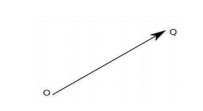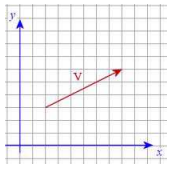# Vectors and their Notations

For students of Physics, there would be plenty of instances where they would have acquainted themselves with terms like vectors, scalars and so on. Here the topic for discussion would be about vectors and their notations.

## What is a Vector?

In most cases, it is necessary that a typical physical quantity has to be provided with magnitude description and direction in a complete manner. For instance, let us take the example of ‘Force’ as it has both direction and magnitude (value in numerical form), and hence a vector quantity. If in case, one provides information like 5 N being the force exerted on a particular object without mentioning the direction of the force, the information would be incomplete. Such is the importance of direction in the case of force as it would turn the information complete. Certain other examples of vector quantities include linear momentum, acceleration and velocity. Therefore, we can define a vector as a quantity having both magnitude and direction.

### How are Vectors Represented?

To denote vectors, arrows are marked above the representative symbols for them. Examples include $$\vec{AB}$$, $$\vec{BC}$$ and so on. Single letters also would be suitable for this purpose like:

• Velocity vector:$$\vec{v}$$
• Force vector: $$\vec{F}$$
• Linear momentum: $$\vec{p}$$
• Acceleration vector: $$\vec{a}$$

#### Vector representation

k . r $$\vec{k}\cdot \vec{r}$$
$$E\times B$$ $$\vec{E}\times \vec{B}$$
$$\bigtriangledown \times r$$
$$\vec{\bigtriangledown} \times \vec{r}$$
v = 0 $$\vec{v}=\vec{0}$$
$$\hat{r}$$ $$\hat{\vec{r}}=\hat{r}$$

## Representation of Two-Dimensional Vectors

Two-dimensional vectors can be represented in three ways as follows:

### Geometric Representation of Vectors

In this vector representation, we use an arrow. The length of the arrow denotes the magnitude of the vector and the direction of the vector is indicated by the arrowhead.Representation of Vector

The vector shown in the diagram can be written as $$\vec{OQ}$$ and its magnitude is written as $$\left | OQ \right |$$.

### Rectangular NotationRectangular Notation of vector

In this type of vector representation, the vector is located in a rectangular coordinate system, as illustrated below.

The rectangular coordination notation for this vector is $$\vec{v}$$ = (6,3). An alternate notation is the use of two unit vectors $$\hat{i}$$ = (1,0) and $$\hat{i}$$ = (0,1) so that $$v=6\hat{i}+3\hat{j}$$

### Polar Notation

In the polar notation, we specify the vector magnitude r, $$r\geq 0$$ and its angle θ with the positive x-axis.

#### Equality of Vectors

If the directions and magnitudes of two vectors are the same, the vectors would be equal in nature. However, one should remember that vectors of the same physical quantity are put into comparison.  For example, it would be feasible to compare the force vector of 5 N in positive x-axis and velocity vector of 5 m/s in the positive x-axis.

From the above discussion, it is obvious that this topic has great significance for students of Physics and would continue to do so in the years to come.

Stay tuned with BYJU’S and fall in love with learning!

Test Your Knowledge On Vector Notations!